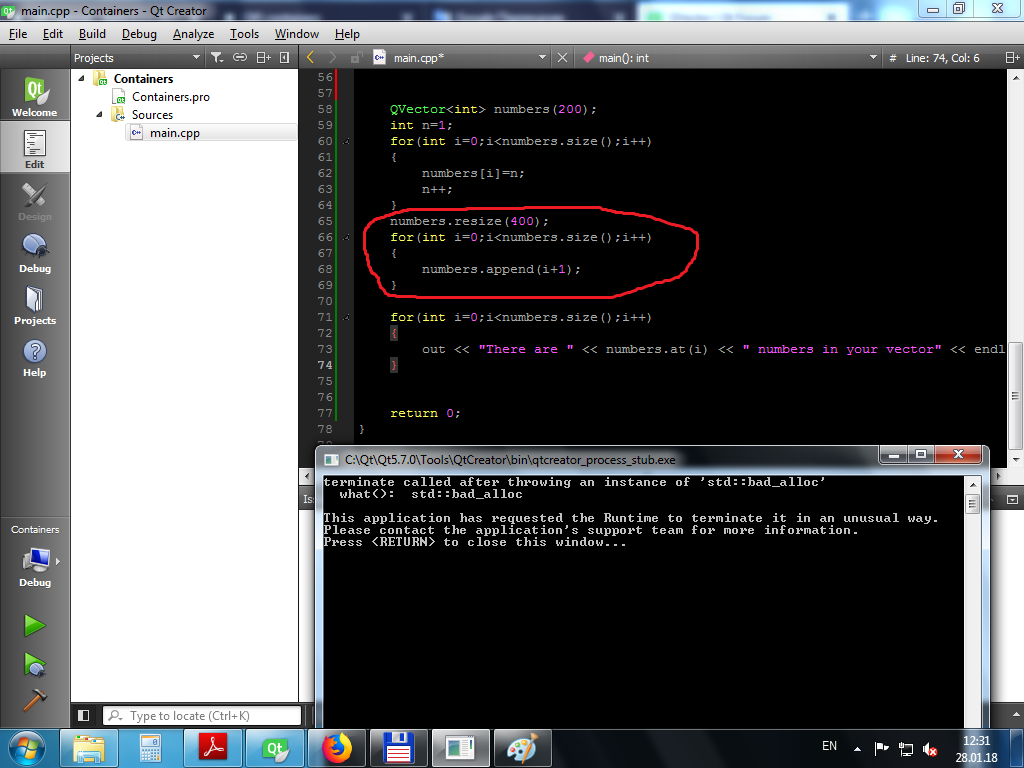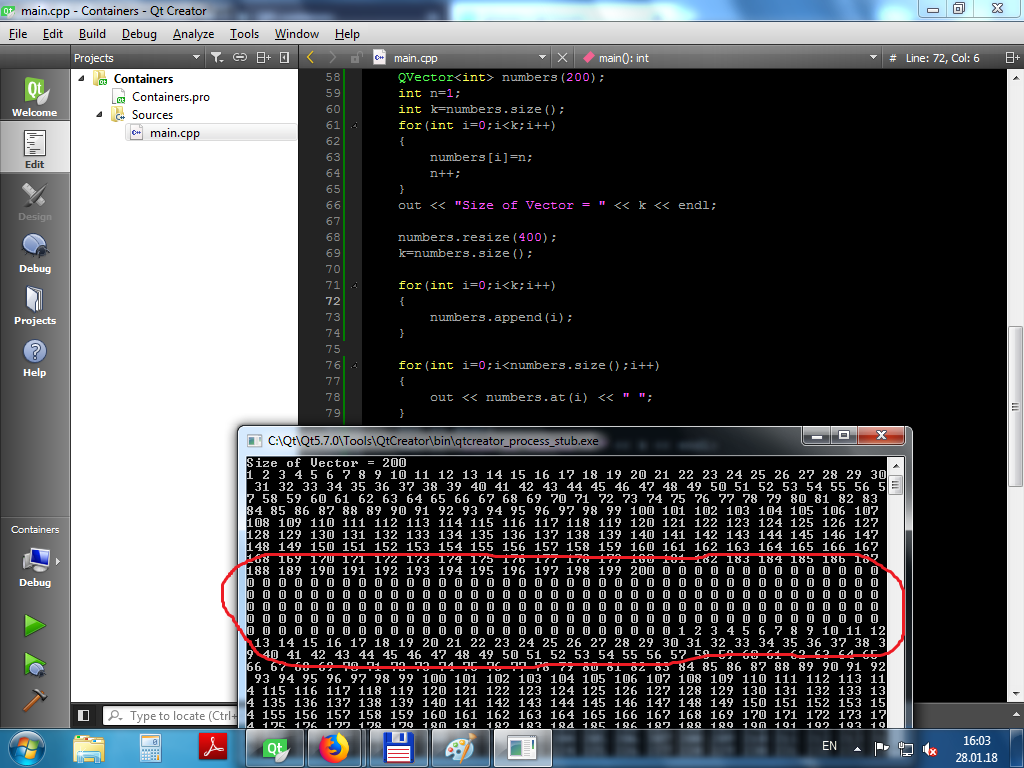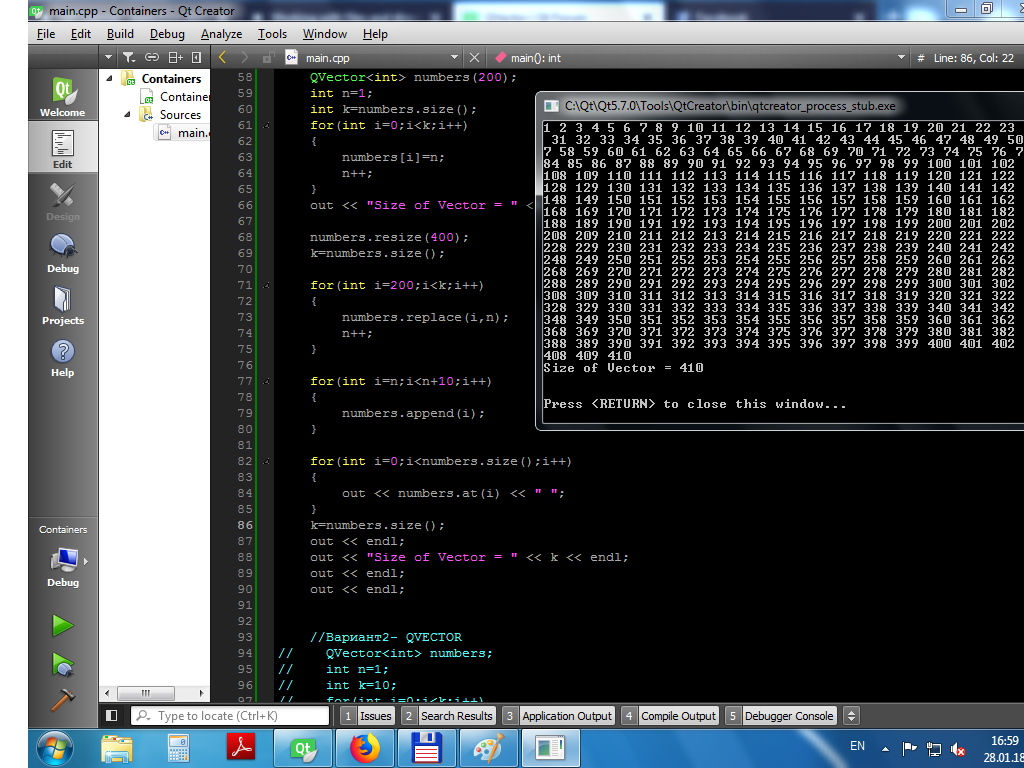# QVector

• Hello all
I am trying to work with QVector and QList. I have two situations.
FIRST:I know the size of vector. Then how to add new items to vector. For Example:
QVector<int> numbers(200);
int n=1;
for(int i=0;i<numbers.size();i++)
{
numbers[i]=n;
n++;
}
this part didn't work properly
numbers.resize(400);
for(int i=0;i<numbers.size();i++)
{
numbers.append(i+1);
}

``````for(int i=0;i<numbers.size();i++)
{
out << "There are " << numbers.at(i) << " numbers in your vector" << endl;
}
``````

***Second***When i didn't know the size of vector when initializing, but i must fill it with numbers later. How to do it?

Regards Jasur,
Tashkent

• What did not work properly ? Look at following documentation. According to my understanding, both the cases you mentioned are achievable. What is the real issue ? What you wanted to achieve ?

``````Sets the size of the vector to size. If size is greater than the current size, elements are added to the end; the new elements are initialized with a default-constructed value. If size is less than the current size, elements are removed from the end.
``````

• @dheerendra

What did not work properly ? In this example:i want to create qvector with 200 elements, then to resize qvector and append new elements to existing qvector. But the part which marked with red didn't work.

What is the real issue ? What you wanted to achieve ?
I want to learn qvector possibilities, not only qvectors but QT. From here i learning QT http://zetcode.com/gui/qt5/containers/.

Regards Jasur,
Tashkent

• @Jasur said in QVector:

QVector<int> numbers(200);

Here, you created a vector with 200 elements.

``````numbers.resize(400);
``````

Here, you resized your vector, so it now has 400 elements.

``````for(int i=0;i<numbers.size();i++)
{
numbers.append(i+1);
}
``````

Each time you call `append()`, you add 1 extra element to your vector. Is this what you want?

@Jasur said in QVector:

But the part which marked with red didn't work.

Look at your code. What is the difference between your first `for` loop and your second `for` loop? Why did you use different code in the 2 loops?

• Hi
The reason the red example crashes is that you
create an infinite loop since you use the size() function as the
stop condition.
In the loop you call numbers.append(i+1) which with add 1 element to list each time.
That means size() will report 400, 401, 402 and so on and the loop never terminates
as i var can never catch up.

If you did

``````numbers.resize(400);
int sizeNow=numbers.size();
for(int i=0;i<sizeNow;i++)
{
numbers.append(i+1);
}
``````

it wont crash and just add 400 new ints to the list.

• @JKSH Yes, you understand right what i want. There are no difference in this 2 loops, they are both do the same , add data to vector, but in second loop i changed code, because i want to learn qvectors functions, to know how to use them, how to work with them. That is why i creating different situations for myself and try to code.

• @mrjj

Where are come from this "0"? What i do wrong?• Hi
You are not doing anything wrong as such
when you do

``````numbers.resize(400); // here it adds 200 new index with the value of zero (default value)
int sizeNow=numbers.size();
for(int i=0;i<sizeNow;i++)
{
numbers.append(i+1); // you ask it to add a new index ( not using the new 200)
}
``````

So the second loops adss 400 new values after the the new 200 added with the resize

There is a huge difference between
numbers.append(i+1);
and
numbers[i]=n;

Append ADDS a new index where as
numbers[i]
accesses a existing index and do not add a new one.
if you use an idex higher than the size of the list, you will crash
numbers = 100; // would crash

• @mrjj Thank you very much mrjj. The last your explanation helped.Now i understand how it all works.
Here what i wanted, the last versionThank you all, too.

• Ok super :)
One last note
the numbers.at() returns a const object so be aware that
you cant use at() to modify the list. only read.

• @mrjj Thank you for attention.

Regards Jasur